# NEETS Module 01 Assignment 3: Direct Current

(Last Updated On: December 8, 2017)

This is the Textbook Assignment: Chapter 3, “Direct Current” from the Module 1 — Introduction to Matter, Energy, and Direct Current in Navy Electricity and Electronics Training Series (NEETS) . If you are looking for a reviewer in Electronics Engineering this will definitely help. I can assure you that this will be a great help in reviewing the book in preparation for your Board Exam. Make sure to familiarize each and every questions to increase the chance of passing the ECE Board Exam.

### Start Practice Exam Test Questions

Choose the letter of the best answer in each questions.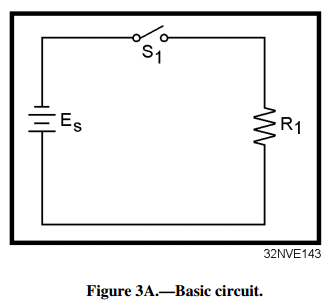Figure 3A.—Basic circuit.

IN ANSWERING QUESTIONS 1 THROUGH 3, REFER TO FIGURE 3A.

1. What parts of the circuit represent the (a) source and (b) load?

• A. (a) Es (b) S1
• B. (a) Es (b) R1
• C. (a) S1 (b) R1
• D. (a) S1 (b) Es

2. Which of the following terms describes the circuit condition?

• A. Partially shorted
• B. Partially open
• C. Shorted
• D. Open

3. Which of the following terms describes the figure 3A?

• A. Parts layout
• B. Exploded view
• C. Wiring diagram
• D. Schematic diagram

4. If circuit voltage is held constant, circuit current will react in what manner as the resistance (a) increases, and (b) decreases?

• A. (a) Increase (b) decrease
• B. (a) Increase (b) increase
• C. (a) Decrease (b) decrease
• D. (a) Decrease (b) increase

5. If circuit resistance is held constant, circuit current will react in what manner as the voltage (a) increases, and (b) decreases?

• A. (a) Increase (b) decrease
• B. (a) Increase (b) increase
• C. (a) Decrease (b) decrease
• D. (a) Decrease (b) increase

3-6. According to Ohm’s law, what formula should be used to calculate circuit voltage if resistance and current value are known?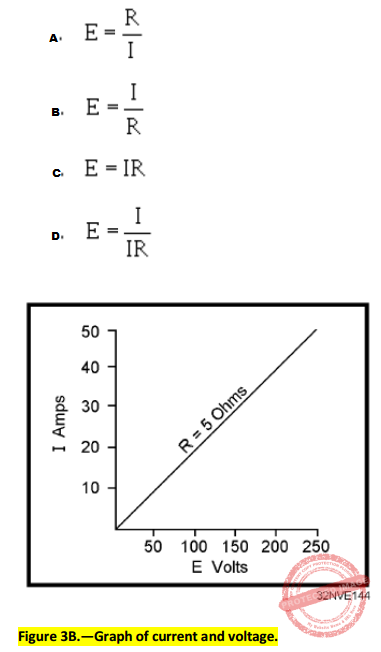Figure 3B.—Graph of current and voltage.

IN ANSWERING QUESTIONS 7 AND 8, REFER TO FIGURE 3B.

7. If the current is 15 amperes, what is the value of the voltage?

• A. 50 V
• B. 75 V
• C. 100 V
• D. 150 V

8. If the voltage is 200 volts, what is the value of the current?

• A. 10 A
• B. 20 A
• C. 30 A
• D. 40 A

9. Which of the following terms applies to the rate at which an electrical force causes motion?

• A. Power
• B. Energy
• C. Inertia
• D. Each of the above

10. Which of the following circuit quantities can be varied ONLY by varying one of the other circuit quantities?

• A. Voltage
• B. Current
• C. Resistance
• D. Each of the above

11. Which of the following is a correct formula for determining power in an electrical circuit?

• A. P = EI
• B. P = I2R
• C. P = E2/R
• D. Each of the above

12. What is the current in a circuit with 15 ohms of resistance that uses 135 watts of power?

• A. 10 A
• B. 15 A
• C. 3 A
• D. 9 A

13. What is the total power used by a 15-ohm resistor with 4 amps of current?

• A. 60 W
• B. 240 W
• C. 360 W
• D. 900 W

14. What type of resistor should be used in question 3-13?

• A. Carbon
• B. Wirewound
• C. Precision
• D. Composition

15. How much total energy is converted by a 1-horsepower motor in 10 hours?

• A. 7.46 kWh
• B. 8.32 kWh
• C. 8.59 kWh
• D. 9.32 kWh

16. If the energy used by the motor in question 3-15 is 9.5 kWh, what is the efficiency of the motor?

• A. 0.981
• B. 0.904
• C. 0.876
• D. 0.785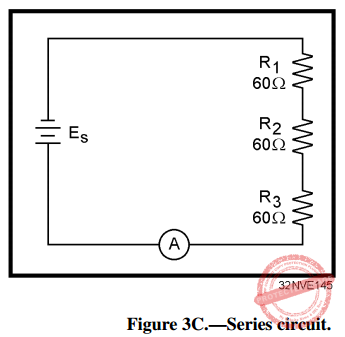Figure 3C.—Series circuit.

IN ANSWERING QUESTIONS 17 THROUGH 23, REFER TO FIGURE 3C.

17. What is the total circuit resistance (R)?

• A. 20 Ω
• B. 60 Ω
• C. 180 Ω
• D. 240 Ω

18. If the circuit current is 3 amps, what is the source voltage (Es)?

• A. 60 V
• B. 180 V
• C. 540 V
• D. 720 V

19. What is the total voltage dropped by each resistor in question 18?

• A. 20 V
• B. 60 V
• C. 180 V
• D. 540 V

20. If the current decreases to 2 amps, what is the total voltage drop across each resistor?

• A. 120 V
• B. 230 V
• C. 310 V
• D. 400 V

21. What would have to be done to the circuit to cause the current to decrease to 2 amps?

• A. The source voltage would have to be increased
• B. The source voltage would have to be decreased
• C. The resistance of R1 would have to be decreased
• D. One of the resistors would have to be removed from the circuit

22. If the circuit current is 2 amps, what is the total power used by each resistor?

• A. 240 W
• B. 460 W
• C. 620 W
• D. 800 W

23. What is the total power used in the circuit if Es = 360 V?

• A. 720 W
• B. 1380 W
• C. 1860 W
• D. 2400 W

24. When Kirchhoff’s voltage law is used to assign polarities to the voltage drop across a resistor, which of the following references is used to indicate the end of the resistor that the current enters?

• A. Ground
• B. Neutral
• C. Negative
• D. Positive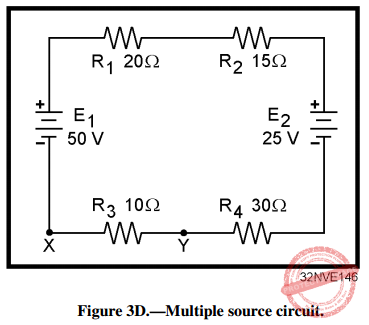Figure 3D.—Multiple source circuit.

IN ANSWERING QUESTIONS 25 AND 26, REFER TO FIGURE 3D.

25. What is the effective source voltage?

• A. 15 V
• B. 25 V
• C. 50 V
• D. 75 V

26. What is the total amount and direction of current through R3?

• A. 1.0 A from Y to X
• B. 1.0 A from X to Y
• C. 0.33 A from Y to X
• D. 0.33 A from X to Y

27. Which of the following terms applies to a circuit in which there is NO complete path for current?

• A. Open
• B. Short
• C. Closed
• D. Grounded

28. A circuit in which the resistance is almost zero ohms is referred to by which of the following terms?

• A. Open
• B. Short
• C. Closed
• D. Broken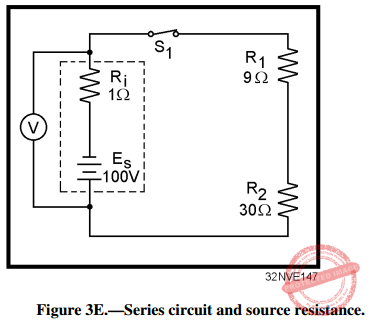Figure 3E.—Series circuit and source resistance.

IN ANSWERING QUESTIONS 29 THROUGH 32, REFER TO FIGURE 3E.

29. If R2 has a short circuit, what will most likely happen to the circuit?

• A. R1 will be destroyed
• B. Es will increase
• C. V will indicate O volts
• D. S1 will automatically open

30. What is the total voltage drop across Ri when the switch is closed?

• A. 2.5 V
• B. 6.5 V
• C. 97.5 V
• D. 100.0 V

31. What will the meter indicate with (a) S1 open, and (b) S1 closed?

• A. (a) 100 V (b) 100 V
• B. (a) 97.5 V (b) 100 V
• C. (a) 100 V (b) 97.5 V
• D. (a) 97.5 V (b) 97.5 V

32. To achieve maximum power transfer in the circuit, which of the following conditions must be met?

• A. Ri = RL
• B. Is = IL
• C. Es = EL
• D. Ks = KL

33. Maximum power is transferred from a source to a load when the value of the load resistance is of what value when compared to the source resistance?

• A. Equal
• B. Twice
• C. One-half
• D. Several times

34. When maximum power is transferred from a source to a load, what is the efficiency of power transfer?

• A. 5%
• B. 25%
• C. 50%
• D. 95%

35. A circuit consists of three resistors connected in parallel. R1= 30 ohms, R2= 15 ohms, and R3= 10 ohms. If the current through R2= 4 amperes, what is the total source voltage?

• A. 20 V
• B. 60 V
• C. 120 V
• D. 220 V

36. What is the relationship of total current to the current through a component in (a) a series circuit, and (b) a parallel circuit?

• A. (a) Divides (b) divides
• B. (a) Divides (b) equals
• C. (a) Equals (b) equals
• D. (a) Equals (b) divides

37. If a current has a negative polarity when Kirchhoff’s current law is applied, which of the following, statements is true of the current?

• A. It is from a battery
• B. It is from a generator
• C. It is entering a junction
• D. It is leaving a junction

38. Three equal resistors are connected in parallel and each resistor has an ohmic value of 300 ohms. What is the equivalent resistance of the circuit?

• A. 100 Ω
• B. 150 Ω
• C. 600 Ω
• D. 900 Ω

39. Three resistors with ohmic values of 120 ohms, 60 ohms, and 40 ohms are connected in parallel. What is the equivalent resistance of the circuit?

• A. 10 Ω
• B. 20 Ω
• C. 30 Ω
• D. 40 Ω

40. Two resistors with ohmic values of 90 ohms and 45 ohms are connected in parallel. What is the equivalent resistance of the circuit?

• A. 10 Ω
• B. 20 Ω
• C. 30 Ω
• D. 40 Ω

41. Which of the following terms describes a single resistor that represents a complex circuit?

• A. Equal resistor
• B. Phantom resistor
• C. Schematic resistor
• D. Equivalent resistor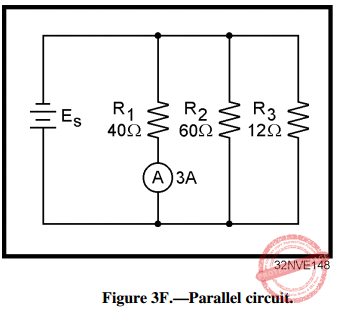Figure 3F.—Parallel circuit.

IN ANSWERING QUESTIONS 42 THROUGH 46, REFER TO FIGURE 3F.

42. What is the value of Es?

• A. 336 V
• B. 300 V
• C. 240 V
• D. 120 V

43. What is the value of current through R2?

• A. 1 A
• B. 2 A
• C. 3 A
• D. 4 A

44. What is the approximate value of total resistance?

• A. 8 Ω
• B. 37 Ω
• C. 112 Ω
• D. 257 Ω

45. What is the value of total power?

• A. 1.2 kW
• B. 1.5 kW
• C. 1.8 kW
• D. 2.0 kW

46. What is the total power consumed by R3?

• A. 108 W
• B. 240 W
• C. 360 W
• D. 1200 W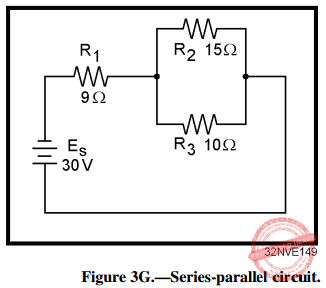Figure 3G.—Series-parallel circuit.

IN ANSWERING QUESTIONS 47 THROUGH 49, REFER TO FIGURE 3G.

47. What is the value of the total resistance?

• A. 3.6 Ω
• B. 15 Ω
• C. 34 Ω
• D. 40 Ω

48. What is the total power used in the circuit?

• A. 22.5 W
• B. 26.5 W
• C. 60.0 W
• D. 250.0 W

49. What is the total voltage drop across R3?

• A. 8 V
• B. 12 V
• C. 18 V
• D. 30 V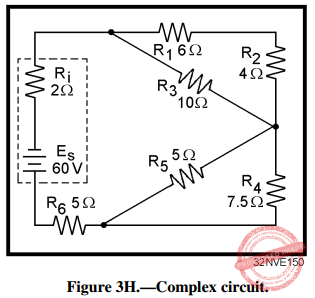Figure 3H.—Complex circuit.

IN ANSWERING QUESTIONS 50 AND 51, REFER TO FIGURE 3H.

50. What is the value of total resistance?

• A. 5 Ω
• B. 8 Ω
• C. 13 Ω
• D. 15 Ω

51. If an equivalent resistor is used to represent the network of R1, R2, R3, R4, R5, and R6, what is the total voltage drop across this resistor?

• A. 8 V
• B. 26 V
• C. 52 V
• D. 60 V

52. If an open occurs in a series portion of a circuit, what is the effect on (a) total resistance, and (b) total current?

• A. (a) Decreases to zero (b) Becomes infinite
• B. (a) Decreases to zero (b) Decreases to zero
• C. (a) Becomes infinite (b) Becomes infinite
• D. (a) Becomes infinite (b) Decreases to zero

53. If an open occurs in a parallel branch of a circuit, what is the effect on (a) total resistance, and (b) total current?

• A. (a) Increases (b) decreases
• B. (a) Increases (b) increases
• C. (a) Decreases (b) decreases
• D. (a) Decreases (b) increases

54. If a short circuit occurs in a series portion of a circuit, what is the effect on (a) total resistance, and (b) total current?

• A. (a) Increases (b) decreases
• B. (a) Increases (b) increases
• C. (a) Decreases (b) decreases
• D. (a) Decreases (b) increases

55. If a short circuit occurs in a parallel branch of a circuit, what is the effect in (a) total resistance, and (b) total current?

• A. (a) Increases (b) decreases
• B. (a) Increases (b) increases
• C. (a) Decreases (b) decreases
• D. (a) Decreases (b) increases

56. If one branch of a parallel network shorts, what portion of the circuit current, if any, will flow through the remaining branches?

• A. An amount determined by the combined resistance of the remaining branches
• B. All
• C. One-half
• D. None

57. Which of the following circuit quantities need NOT be known before designing a voltage divider?

• A. The current of the source
• B. The voltage of the source
• C. The current requirement of the load
• D. The voltage requirement of the load

THE FOLLOWING INFORMATION IS TO BE USED IN ANSWERING QUESTIONS 58 THROUGH 60: A VOLTAGE DIVIDER IS REQUIRED TO SUPPLY A SINGLE LOAD WITH +150 VOLTS AND 300 MILLIAMPS OF CURRENT. THE SOURCE VOLTAGE IS 250 VOLTS. (HINT: DRAW THE CIRCUIT.)

58. What should be the value of the bleeder current?

• A. 3 A
• B. 300 mA
• C. 30 mA
• D. 3 mA

59. What should be the ohmic value of the bleeder resistor?

• A. 50
• B. 500
• C. 5 kΩ
• D. 50 kΩ

60. What is the value of total current?

• A. 303 mA
• B. 330 mA
• C. 600 mA
• D. 3300 mA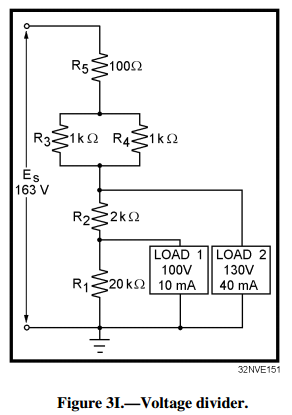Figure 3I.—Voltage divider.

IN ANSWERING QUESTIONS 61 THROUGH 66, REFER TO FIGURE 3I.

61. Why must the value of R1 be calculated first?

• A. For convenience
• B. The current through R2 depends on the value of R1
• C. The voltage drop across R1 depends on the value of load 1
• D. In any circuit, values for resistors labeled R1 are calculated first

62. How is the current through R2 calculated?

• A. By adding IR1 and the current requirement of load 1
• C. By subtracting the current requirement of load 1 from the current requirement of load 2
• D. By subtracting the current requirement of load 2 from the current requirement of load 1

63. How is the voltage drop across R2 calculated?

• B. By subtracting the voltage drops across R5 and R3 from the source voltage
• C. By subtracting the voltage requirement of load 1 from the voltage requirement of load 2
• D. By subtracting the voltage requirements of load 1 and load 2 from the source voltage

64. What is the minimum wattage rating required for R5?

• A. 1 W
• B. 2 W
• C. 1/2 W
• D. 1/4 W

65. What is the total power supplied by the source?

• A. 3.765 W
• B. 7.965 W
• C. 8.209 W
• D. 8.965 W

66. What is the purpose of using the series-parallel network consisting of R3, R4, and R5 in place of a single resistor?

• A. It provides the desired resistance with resistor values that are easily obtainable
• B. It provides the close tolerance required for the circuit
• C. It is more reliable than the use of a single resistor
• D. It costs less by using three resistors of lower wattage rating than a single, large power resistor

67. A single voltage divider provides both negative and positive voltages from a single source voltage through the use of a

• A. ground between two of the dividing resistors
• B. ground to the positive terminal of the source
• C. ground to the negative terminal of the source
• D. ground to the input of all loads requiring a negative voltage

68. Which of the following voltages are considered dangerous?

• A. Voltages above 115 volts only
• B. Voltages above 230 volts only
• C. Voltages above 450 volts only
• D. All voltages

69. If you discover a possible malfunction in an electric circuit, which of the following actions should be taken?

• A. Attempt repairs yourself
• B. Report the malfunction to a qualified technician
• C. Ignore the malfunction unless you were assigned to repair it
• D. Secure the circuit immediately by removing power at the nearest switch

70. If a person has stopped breathing and there is NO detectable heartbeat, who should perform CPR?

• A. Medical personnel only
• B. The first person on the scene
• C. Emergency Medical Technicians only
• D. Trained, qualified personnel only

Help Me Makes a Difference!

 P inoyBIX educates thousands of reviewers/students a day in preparation for their board examinations. Also provides professionals with materials for their lectures and practice exams. Help me go forward with the same spirit. “Will you make a small \$5 gift today?” Option 1 : \$1 USD Option 2 : \$3 USD Option 3 : \$5 USD Option 4 : \$10 USD Option 5 : \$25 USD Option 6 : \$50 USD Option 7 : \$100 USD Option 8 : Other Amount© 2014 PinoyBIX Engineering. © 2019 All Rights Reserved | How to Donate? |#### GEAS Solution

Dynamics problem Economics problem Physics problem Statics problem Strength problem Thermodynamics problem

#### Questions and Answers in GEAS

Engineering Economics Engineering Laws and Ethics Engineering Management Engineering Materials Engineering Mechanics General Chemistry Physics Strength of Materials Thermodynamics
Consider Simple Act of Caring!: LIKE MY FB PAGE

Our app is now available on Google Play, Pinoybix Elex